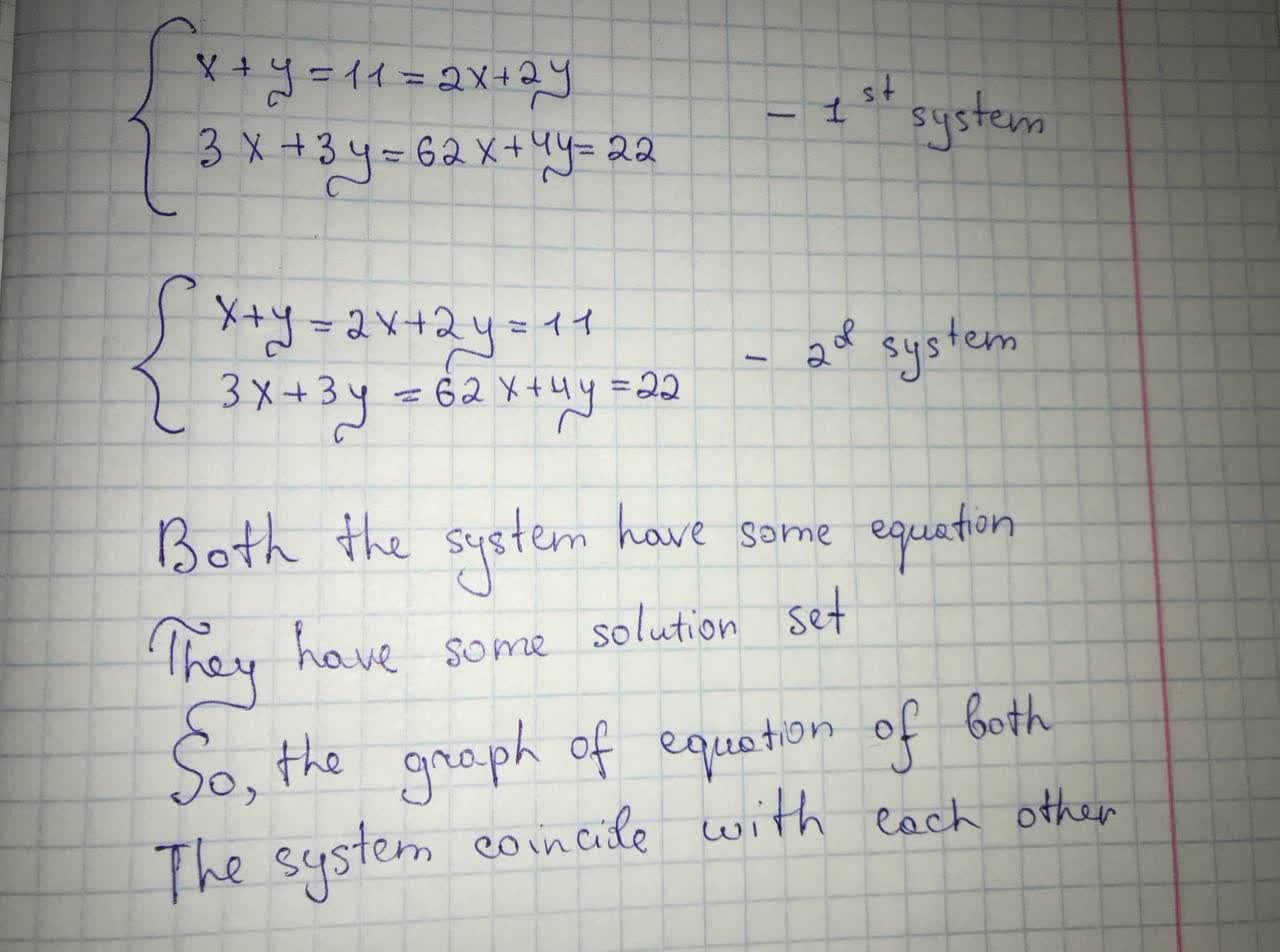Phoebe

2021-08-29

$\left\{\begin{array}{l}x+y=2x+2y=11\\ 3x+3y=62x+4y=22\end{array}$
$\left\{\begin{array}{l}x+y=2x+2y=11\\ 3x+3y=62x+4y=22\end{array}$
Two systems of equations are shown.
Which TWO of the following statements each provide sufficient reasoning to show that the systems have the same solution?nitruraviX

Given that:Do you have a similar question?